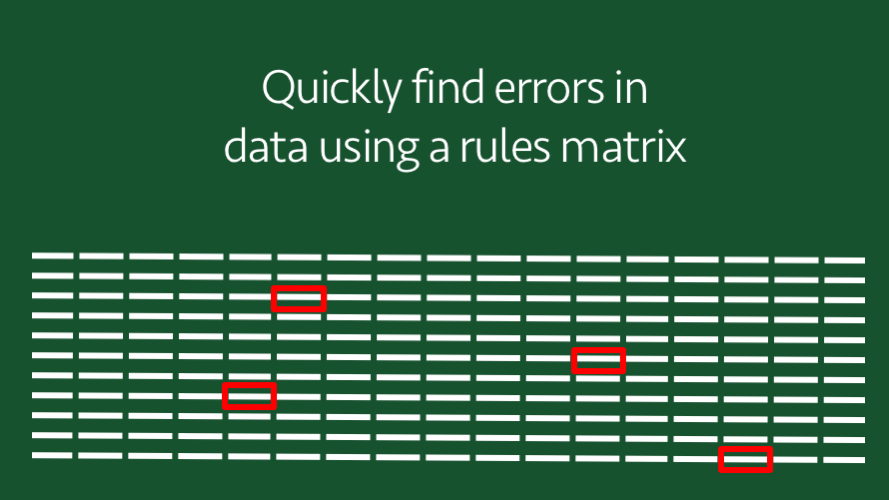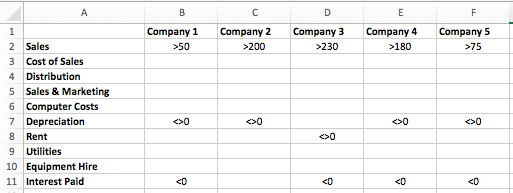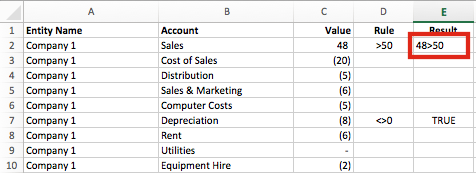# Quickly find errors in data using a rules matrixBeing able to analyze a lot of data quickly to find potential anomalies and errors is a skill.  We should not assume that the data we use in our spreadsheets is error free.  To make this assumption might be dangerous, especially if we are providing insights and advice based on that data.  But in a world of too much data how can we do this quickly and efficiently?  Today, I want to share one method with you.  I want to show you how to create and apply a rules matrix.

## What is a rules matrix?

A rules matrix is a grid showing the validation rules which we want to apply to our data.  Look at the screenshot below.In this example, we have 5 companies and 10 accounts which we might find within a profit statement.  This simple matrix contains the rules we wish to apply, for example:

• Sales for Company 1 must be above 50
• Rent for Company 3 must not be zero
• Interest Paid for Company 4 must be less than zero

We could set a rule for every account and every company in the matrix, or we could just apply the rules to specific cells.  When we compare our data to these rules we have a very fast way of checking our data for obvious errors.

## How to use the rules matrix?

The screenshot below shows a worksheet with the Entity Name (column A), Account (column B) and Value (column C).  This is the type of data which could come straight from an accounting or ERP system.Notice that in cell E1, FALSE is displayed in red, this is indicating that the cell C2 has failed the rule stated in Cell D2.  Company 1 has Sales of 48, which is less than the 50 stated by the rule.  Company 1 has a Depreciation cost of -8 (cell C7), this passes the rule in cell D7, so cell E7 displays TRUE.

Each period we could update columns A, B and C with new data.  The rules would then be applied automatically, so we can identify the error and anomalies.

The workbook for this example is available for download here.

## Retrieve the rule from the Rules worksheet

Let’s look at the magic which enables this to happen.

Our workbook has two worksheets, Data (containing the list of Companies, Accounts and Values) and Rules (containing the rules matrix)

Firstly, let’s consider cell D2 of the Data worksheet.  This cell retrieves the rule from the rules matrix.

`=INDEX(Rules!\$B\$2:\$F\$11,MATCH(Data!\$B2,Rules!\$A\$2:\$A\$11,0),MATCH(Data!\$A2,Rules!\$B\$1:\$F\$1,0))&""`

All the populated cells in the rules matrix contain a symbol at the start (e.g. bigger than: >, not equal to: <>), Excel assumes this to be a text string.  But Excel also assumes that a blank cell has a value of zero, rather than an empty text string.  We can convert this zero to an empty string by adding &”to the end of the formula.

`=INDEX(Rules!\$B\$2:\$F\$11,MATCH(Data!\$B2,Rules!\$A\$2:\$A\$11,0),MATCH(Data!\$A2,Rules!\$B\$1:\$F\$1,0))&""`

Provided we have entered the \$ signs in the correct place we can copy this formula down to the bottom of our data.

## Compare the rule to the value

Now, let’s consider cell E2 of the Data worksheet.  This cell compares the value of cell C2 with the rule in cell D2.  This should be easy, right?  Using normal logical operators =48>50 would always show a FALSE.  However, cell C2 is a number and cell D2 is a text string.  If we tried to combine the cells we could enter =C2&D2 in cell E2.  But this will return this:Hmmm . . .  not quite what we wanted.  We want it to show TRUE/FALSE, but instead, it displays 48>50 as text.

In a previous post, I shared how to convert a text string into a formula, this is the technique we need to apply here.

If we insert the following VBA code into a Module of the Visual Basic Editor we can create our own EvaluateString() formula, which will convert a text string into a formula.

```Function EvaluateString(Ref As String)
Application.Volatile
EvaluateString = Evaluate(Ref)
End Function```

Note: If you do not know how to insert VBA code into a module consider following my Beginning VBA course.

Once we have entered the VBA code we can enter the following formula into cell E2.

`=EvaluateString(C2&D2)`

This formula will display TRUE if the value meets the rule, or FALSE if the value fails the rule.  In Cell E2, 48 is not bigger than 50, so it will display FALSE.

We can now copy this formula down to the bottom of the data.

## The final touches

In my example workbook, the last thing I did was to use conditional formatting to highlight the FALSE values red.  If there is a lot of data, you could also apply a filter to show the FALSE results.  I have left this example as a simple TRUE/FALSE, but you could easily change the TRUE/FALSE result to display something else.

## Using the rules matrix in a real scenario

It takes a bit of work to set-up the rules matrix, but once it is working it can be a significant time saver.  In our example, we only have 10 accounts and 5 companies, but it works equally well with hundreds of accounts and hundreds of companies, therefore you can analyze thousands of rows of data in just a few seconds.  Hopefully, you will agree that this is a good example of Excel doing the work for you.

## Want to Learn VBA & Macros?

If you want to automate Excel and save time, my recommended resouces for learning VBA Macros are:

### Books:

Excel 2016 Power Programming with VBA